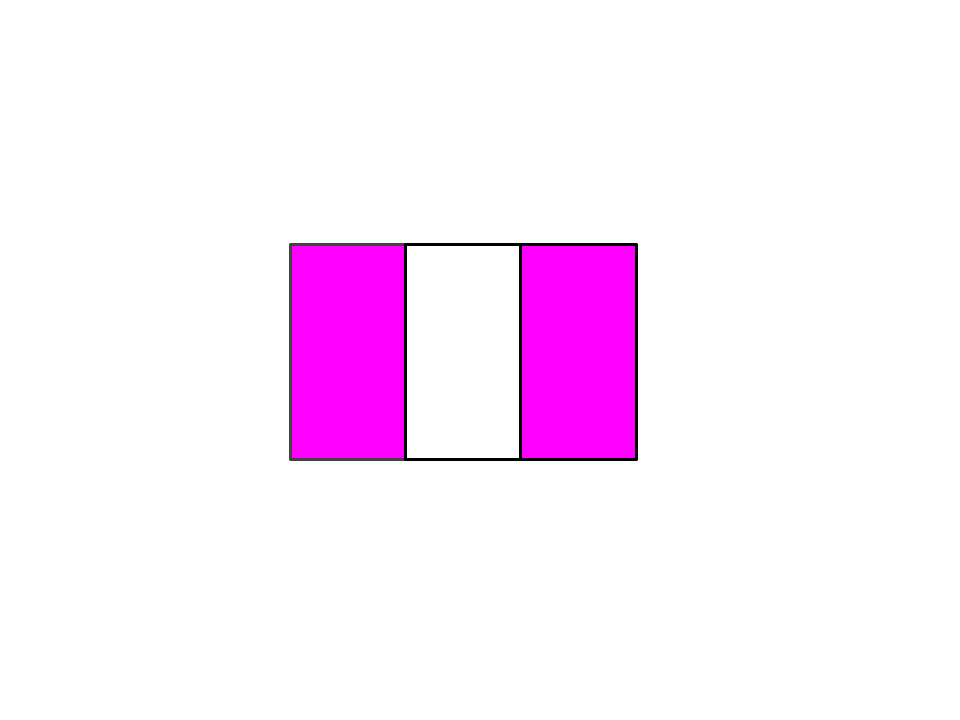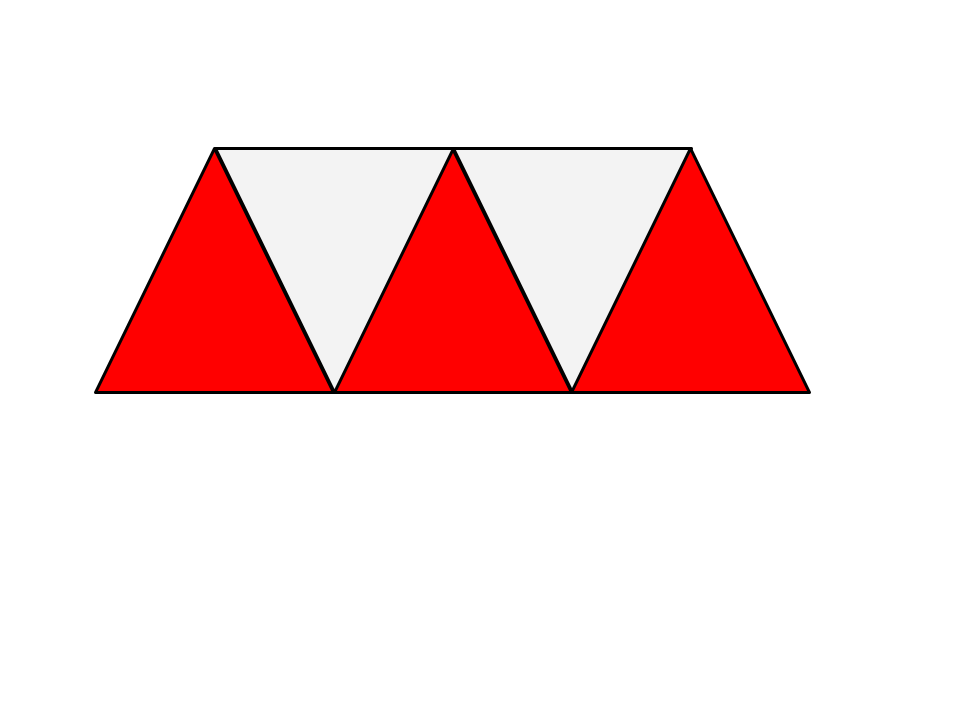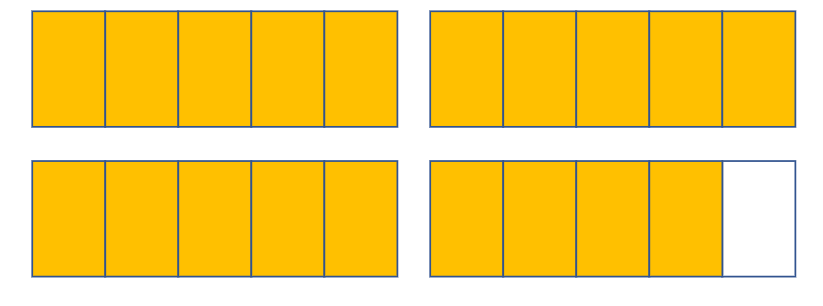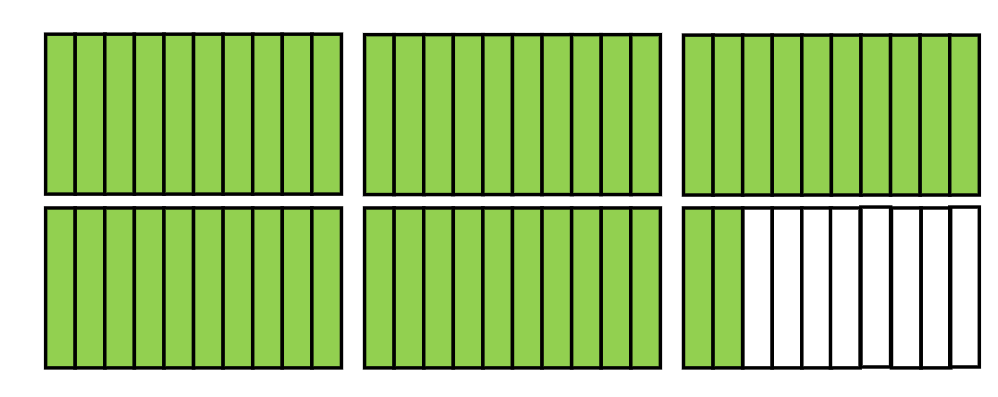Identifying fractions
Compare and Order Fractions
Find an equivalent fraction
Multiplying Fractions
Vocab!
Mixed
1001/2

100

Compare the fractions using <, >, or =

4/8     2/4

4/8  =  2/4

100

1/2 by multiplying by 2

2/4

100

1/2 x 2

2/2 or 1

100

The top number in a fraction is called the?

Numerator

100

Mr. Cosentino cut his pizza into 8 slices, and ate two of them. What fraction of the pizza did he not eat?

6/8

2002/3

200

Compare the fractions using <, >, or =.

3/4     3

3/4  <  3

200

2/3 by multiplying by 3

6/9

200

4/5 x 3

12/5 or 2 and 2/5

200

Two fractions which name the same value are called?

Equivalent fractions

200

Find an equivalent fraction for 1/4

3003/5

300

Compare the fractions using <, >, or =.

1/2     1/4

1/2 > 1/4

300

3/4 by multiplying by 3

9/12

300

3/4 x 8

24/4 or 6

300

The bottom number in a fraction is called the?

Denominator

300

Multiply:

3/5 x 3

9/5 or 1 and 4/5

4003 and 4/5

400

Compare the fractions using <, >, or =.

2/4   2/10

2/4 > 2/10

400

2/5 by multiplying by 2 then again by 2

8/20

400

1 and 1/3 X 2

8/3 or 2 and 2/3

400

What is it called when we have a whole number and a fraction?

A mixed #

400

Multiply:

3 and 1/2 x 4

12 and 4/2 OR 14

5005 and 2/10

500

Compare the fractions using >, <, =

1/2     3/6

1/2 = 3/6

500

7/8 by x 8

56/64

500

2 and 3/4 x 8 = ?

22

500

When do we use fractions in real life?

Measuring things with a tape measure.

Cooking (3/4 cup of sugar).

Dividing items between people.

Etc.

500

multiply: 6 x 3/4

4 1/2

Click to zoom